# AP Board 8th Class Physical Science Solutions Chapter 1 Force

AP State Syllabus AP Board 8th Class Physical Science Solutions Chapter 1 Force Textbook Questions and Answers.

## AP State Syllabus 8th Class Physical Science Solutions 1st Lesson Force

### 8th Class Physical Science 1st Lesson Force Textbook Questions and Answers

Question 1.
What is a force? What changes can be produced by a force?
Force: A push or a pull of an object is called a force.
Changes produced by a force:

1. A force can change the state of motion of an object.
2. A force can change the shape of an object.

Question 2.
State an example for a situation mentioned below in which a force:
a) Changes the speed of an object.
b) Changes the shape of an object.
c) Changes the direction of an object.
a) Force changes the speed of an object:
A ball at rest begins to move when a force is applied on it that shows force changes the speed of an object.
b) Force changes the shape of an object:
Take a plastic or metal scale placed between two bricks and put some weight at the centre of the scale. We observe that force changes its shape.
c) Force changes the direction of an object:
Kick a moving football with some angle then we can observe the change in the direction of football.Question 3.
How can you differentiate between a contact force and a force at a distance?
(OR)
Distinguish between a contact force and a force at a distance.

 Contact force A force at a distance 1. Force which results when there is a direct physical contact between two interacting objects is known as contact force. 1. The force which occurs without any physical contact between two objects is known as a force at a distance or field force. 2. The two objects have direct contact. 2. No contact. 3. For example, the tyres of a bicycle has direct contact with the surface of the road. 3. For example, the attraction of two magnets.

Question 4.
Give two examples each for a contact force and a force at a distance.
Contact force:
e.g.: 1) Muscular force 2) Frictional force Force at a distance:
e.g.: 1) Magnetic force 2) Electrostatic force

Question 5.
Detect the errors in the following statement and rewrite it making necessary corrections.
“Because the car is at rest, no forces are acting on it.”
The object is said to be at rest when no forces are acting on it or net force acting on it is zero. So the statement should be corrected like this “Because the car is at rest, no forces are acting on it or net force on the car is zero.”

Question 6.
Why do tools meant for cutting always have sharp edges?
The tools meant for cutting have sharp edges because of smaller contact area they exerts more pressure on objects and cutting of objects are easy.

Question 7.
Objects change their state of motion due to the net force acting on them. Do you agree with this statement ? Discuss with examples.
Yes, we can agree with this statement. Net force can increase or decrease the speed of an object. Force can also change the direction of an object.
Hence we conclude that net force can change the state of motion of an object.
Some examples:

1. If we kick the ball it will move from state of rest.
2. If we stop the running ball it will come to state of rest.
3. If we through a ball on the ground it will come to rest after sometime due to gravitational force acting on it.
4. If we pull the chair, it will move due to muscular force acting on it.Question 8.
When you push a heavy object, it doesn’t move. Explain the reason in terms of net force.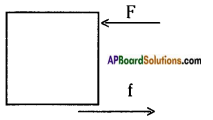When we apply force on a heavy object it has to overcome frictional force in order to move. If the force applied is less than the frictional force then the net force (f – F) is insufficient to move the object.

Question 9.
Find net forces from the following diagrams: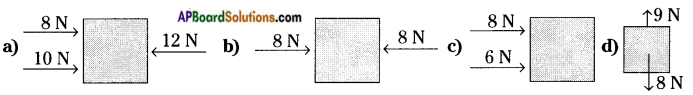a) Net force is 6N along horizontal direction towards right.
b) Net force is zero.
c) Net force is 14N along horizontal direction towards right.
d) Net force is 1 N upwards in vertical direction.

Question 10.
Imagine that friction has disappeared from the earth. What will happen? Explain.
If friction has disappeared from the earth then

1. we cannot walk or run.
2. we will not have any cars or bicycles because all of them move due to friction.
3. no building could be constructed.
4. carpenters will not able to smoothen surfaces.
5. we could not fix nail on the wall.
6. it will not possible to write with pen or pencil.
7. we will not able to hold any appliances such as hammer, soap, etc.Question 11.
Karthik is observing the live telecast of a one day cricket match. He noticed motion of a roller on the pitch during lunch break. He thought about various forces acting on the roller and the net force when it is in motion. Many questions arose in his mind regarding the direction of the net force. Can you guess what would be those questions?

1. Why there is no motion for roller in vertical direction?
2. What are the forces acting in the vertical direction?
3. What are the forces acting in the horizontal direction?
4. Why there is motion in the horizontal direction for roller?

Question 12.
a) Take two identical straws and suspend one of them freely. Rub the other straw with a piece of paper. Bring the rubbed end of the straw near the suspended one. What do you observe from this activity? Can you tell which type of force is it?
b) Comb your dry hair. Bring the comb near small pieces of paper. What do you observe? Explain.
a) The rubbed end of the straw attracts the suspend straw when it is brought near to it. The reason is that when the straw is rubbed with a paper, it acquires an electrostatic charge on its surface and the suspended straw near it acquires opposite charge.
b) When we comb dry hair and bring small pieces of paper they are attracted by comb the reason is when we comb dry hair, it acquires electrostatic charge and paper pieces brought near to it acquire opposite charge there by they are attracted by comb.

Question 13.
Collect pictures from various sources like internet, magazines, newspapers, etc. to illustrate contact forces, forces and prepare a scrap book.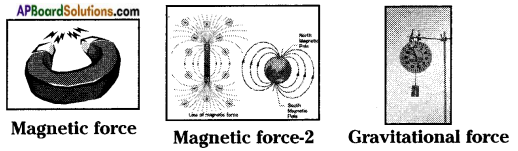Question 14.
A stick is placed on steps as shown in the figure. Draw normal forces on the stick.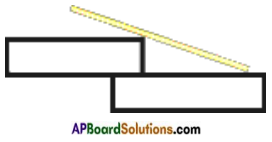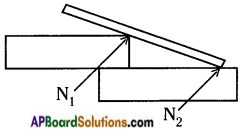Question 15.
A monkey hangs stationary at the end of the vertical vine. What forces act on the monkey
a) The weight of the monkey downward direction.
b) The normal force by branch upward direction.
c) The frictional force exerted by branch along the horizontal direction.Question 16.
If you push on a heavy box which is at rest, you must exert some force to start its motion. However, once the box is sliding, you can apply a lesser force to maintain that motion. Why?
When the box is sliding, the contact points on its surface do not get enough time to lock in to the contact points on the floor. So friction is less when compared with box at rest so we required to apply lesser force to maintain motion.

Question 17.
How do you increase the pressure by keeping
(a) area unchanged and (b) force unchanged?
Pressure P = hdg. So pressure can be depend on height of air column, density of air and acceleration due to gravity.
So pressure can be increased by increasing length of air column at a particular place and for a particular gas.

Question 18.
Design and conduct experiments to test few ways how friction may be reduced.
Friction is due to the roughness of surfaces. It can be reduced by the following methods.
– By polishing the rough surface.
– By applying lubricants like oil and grease to the surfaces.
– By spreading powder on the rough surface like carromboard.
– By using soap and detergent solutions, etc.
Experiment: The rusted pulley makes a rattling noise. Apply some oil in between the wheel and axle. The sound is reduced and now it works smoothly.

Question 19.
Observe the figure and find in which direction the force of friction acts. Also indicate the normal forces and their directions.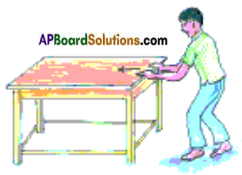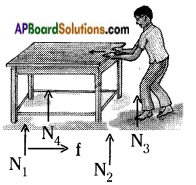f: force of friction
N1, N2, N3, N4 are normal forces.Question 20.
A man is standing still on a level floor. What forces act on him? Draw a free body diagram (FBD) to show all forces acting on him.
The forces acting on the man: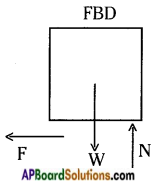Along vertical direction:

1. Weight of the man or gravitational force acting vertically downwards.
2. Normal force or reaction applied by the floor vertically up.

Along horizontal direction:
Frictional force applied by the floor on the man.

Question 21.
How do you appreciate the role of friction in facilitating our various activities?
Every motion that occurs on earth depends upon friction. For example, we are unable to walk if there is no friction and we cannot write with a pen and we cannot construct a building and there should be no vehicles without friction.
So without friction there is no motion on earth. So friction is required for better living of human beings.

### 8th Class Physical Science 1st Lesson Force InText Questions and Answers

8th Class Physical Science Textbook Page No. 3

Question 1.
When an object slips off your hand, it always falls down. What pulls it down?
Gravitational force.

Question 2.
If you roll a ball on a level ground, it slows down and after sometime it will come to a stop. What makes the ball stop?
Frictional force acting between floor and ball.

Question 3.
What forces acting on objects, change their state or state of motion?
Contact force and force at a distance.

Question 4.
Why does the needle of a magnetic compass move when we place a bar magnet near to it?
A force at a distance or field force acts on the needle. So, the needle of a magnetic compass moves when we place a bar magnet near it.Question 5.
Why does the toothpaste come out when we press the tube?
A contact force acts between our hand and tube. So, the toothpaste comes out when we press it.

Question 6.
Have you observe the difference between the force applied by a magnet on the needle of a compass and the force you applied on the tube?
First one is force at a distance and the second one is contact force.

8th Class Physical Science Textbook Page No. 4

Question 7.
If a pen slips off from our hands it falls down to the floor.
a) Why does the pen fall down ?
b) What is the force which pulls the pen down ?
a) Due to increase of gravitational pull of the earth than the normal force,
b) Gravitational pull of the earth.

Question 8.
If we keep the pen on a table, it does not fall down. Why ?
As the normal force and gravitational pull of the earth are equal, it does not fall down.

Question 9.
a) Why does a stone thrown up into the sky fall back to the earth?
b) Why do rivers flow down to the sea?
c) How does the earth hold the atmosphere?
d) Is there any force pulling the objects towards earth?
For all the above questions, the answer is ‘due to gravitational pull of the earth on the nearer bodies of the earth’.

8th Class Physical Science Textbook Page No. 6

Question 10.
In all the actions that we perform in our daily life like brushing, bathing, eating, writing, driving and walking ; we have to exert a force. Do you know from where the force comes?
The force comes from our muscles.

8th Class Physical Science Textbook Page No. 8

Question 11.
Did you ever experience slipping on a floor? What conditions caused you to slip?
Yes, it was due to less friction between my foot and floor.Question 12.
Did you experience slipping while you are walking on wet mud ? Why do most of road accidents happen during rainy days?
Yes, in the wet mud the friction is very less and wet mud acts as a lubricant between our feet and floor. During rainy season the mud or road will be wet and as mentioned above the friction will be less, so most accidents happen.

Question 13.
Would it be possible to drive a car if there were no friction between the tyres and the road?
No, we can’t stop the car as there is no friction in case of no friction between the tyres of car and the road. We can drive, but the car would not get any control.

Question 14.
Place your science book on the table. Is it in a state of rest?
Yes.

8th Class Physical Science Textbook Page No. 9

Question 15.
What is the state of the stone?
At rest.

Question 16.
What forces are acting on it?
Tension and gravitational pull of the earth.

Question 17.
What will happen if the thread is broken?
The gravitational pull of the earth increases and the stone falls down.Question 18.
What do we call this force ? (the force supporting the stone)
Tension.

8th Class Physical Science Textbook Page No. 13

Question 19.
You might have seen children playing with a rubber tyre by pushing it with a stick.
They push the tyre again and again with the stick to increase its speed,
a) Do you understand why the speed of the tyre increases whenever it is pushed by the stick?
With every push they are applying a little more force on the moving tyre in the direction of motion. Hence the speed of the tyre increases continuously.
b) Give some more examples where the object speeds up or slows down or a change may occur in its direction of motion, when we exert a force on it?
If wind blows in the direction of motion of bicycle the speed of bicycle increases. If wind blows in the opposite direction of motion of bicycle the speed of bicycle decreases.

Think and Discuss

8th Class Physical Science Textbook Page No. 5

Question 1.
A cricket ball of mass’m’ is thrown upward with some initial speed. If the air resistance is neglected, what forces are acting on the ball when it reaches (a) half its maximum height and (b) its maximum height?
The forces which are acting on the ball thrown vertically upward:
a) At half of its maximum height:

1. The remaining force which acts vertically upwards (some force that we applied is utilized in reaching half of maximum height).
2. The gravitational force downwards.

b) At maximum height:
Gravitational force acts downward direction (The applied force is completely utilized in reaching maximum height).

8th Class Physical Science Textbook Page No. 6Question 2.
Two identical bars, one which is steel and the other a magnet, are painted with the same colour. How can you tell which one is the magnet using only these two bars? (don’t break the bars)
The bar which attracts the other bar is magnet. The other bar is magnetic material that is steel.

8th Class Physical Science Textbook Page No. 8

Question 3.
A book placed on a table is at rest. Is the force of friction acting on it or not ? Explain.
Friction is caused by the irregularities on the two surfaces in contact. Even those surfaces which appear very smooth have large number of minute irregularities on them. Irregularities on the two surfaces lock into one another. So a book placed on a table is at rest experiences frictional force.

8th Class Physical Science Textbook Page No. 10

Question 4.
A system of two bodies A and B are placed as shown in figure. How many forces are acting on A and B respectively?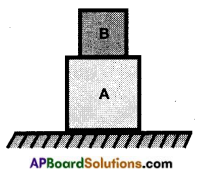On A

1. Normal force exerts by floor vertically upwards.
2. Weight of A vertically downwards.
3. Frictional force exerts floor along horizontal direction.

On B

1. Normal force exerted by surface of A vertically upwards.
2. Weight of B vertically downwards.
3. Frictional force exerts by surface of A along horizontal direction.

Question 5.
Why is it necessary to separate contact force into a normal force and frictional force? Give at least two reasons.
Reasons:

1. In order to know what are the forces acting on the object.
2. To know the net force acting on the body.
3. To know the direction of motion of an object.

8th Class Physical Science Textbook Page No. 12Question 6.
Play arm wrestling with your Mend. How can you explain the winning of the game by using the concept of net force?
The person we exerts more muscular force tends to win.

Question 7.
Name the forces acting on arm and their direction while playing the game. Try to draw FBD for this situation.
The muscular force, normal force and weight (gravitational force).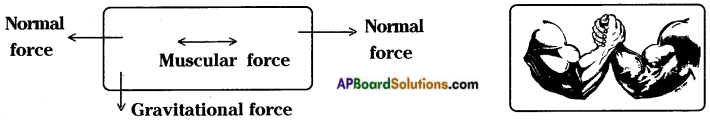8th Class Physical Science Textbook Page No. 15

Question 8.
Does pressure have direction? Explain.
Pressure exerts in all directions. So there is no specified direction for pressure. So we does not consider pressure has direction i.e., it has only magnitude but not direction. So it is a scalar quantity.

8th Class Physical Science 1st Lesson Force Activities

Activity – 1

Question 1.
Identifying push or puli:
Write push or pull in the blank boxes given below. If you feel that the action involves both push and pull, write both in the boxes.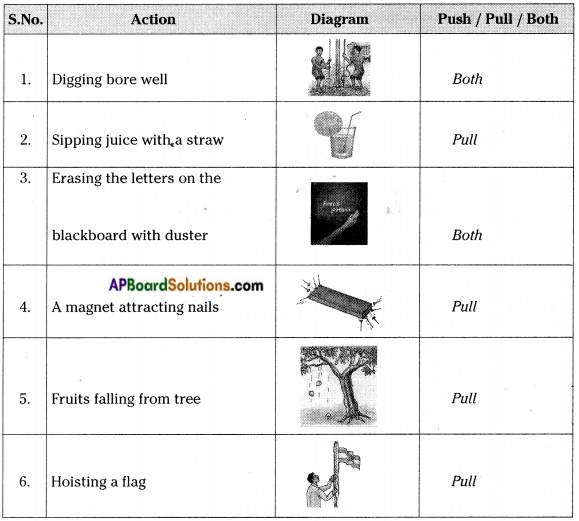a) List three more activities where we exert force which appears as a push.

1. Pushing a repaired car.
2. A football player taking a penalty kick.
3. A cricket ball hit by a batsman.

b) List three more activities where we exert a force as a pull.

1. Drawing bucket of water from a well.
2. Opening a drawer.
3. In a game of tug of war, two teams pull the rope in the opposite directions.

c) State three actions which involve both push and pull.

1. Moving a book front or back on a table.
2. Opening or shutting a door.
3. Cleaning the floor with a cloth.

d) Shall we call the effort done on an object by means of pushing or pulling as a force exerted on the object?
Yes, it is called force.

e) When an object slips off your hand, it always falls down. What pulls it down?
Gravitational force.f) If you roll a ball on a level ground, it slows down and after sometime it will come to a stop. What makes the bail stop?
Frictional force acting between floor and ball.

g) What forces acting on objects, change their state or state of motion?
Contact force and force at a distance.
Types of forces:

h) Why does the needle of a magnetic compass move when we place a bar magnet near it?
Due to magnetic force of magnet.

i) Why does the toothpaste come out when we press the tube?
Due to muscular force applied by us on toothpaste.

j) Have you observe the difference between the force applied by a magnet on the needle of a compass and the force you applied on the tube?
First one is force at a distance and the second one is contact force.

Activity – 2

Question 2.
Observing the magnetic force :
Take a sewing needle. Rub it with a bar magnet several times always moving the magnet in the same direction.
a) Does the needle get magnetised?
Yes. Identify south and north pole of the magnetised needle. Pin a red coloured foam ball to South pole and white ball to North pole of the needle, then drop it in a bowl of water it floats.
Make another needle in the same way. Float both the needles side by side such that like ends facing each other.

b) What happens to the needles? How do they move?
They repel each other. They move backwards to each other.

c) Now place the needles in such a way that unlike ends face each other. How do they push or pull each other?
They pull each other that means they are attracted.

d) What is this pull or push between two poles called?
Magnetic force.

Activity – 3

Question 3.
Observing electrostatic forces :
Take a balloon. Inflate it and tie up the open end. Now cut a paper into small pieces and place them on floor. Rub the balloon with a paper and using the balloon near the pieces of the paper.
a) Are the bits of paper pulled towards the balloon?
Yes, the bits of papers pulled towards balloon.

b) Why does the balloon pull or attracts the pieces of paper?
When the balloon is rubbed with a paper it acquires charge and it induces opposite charge which brought near to it. So the pieces of paper are attracted by balloon.

c) Try to use pepper and salt in the place of pieces of paper. What do you observe?
They do not attracted by the balloon.
Gravitational force: It is our common experience that if a pen slips off from our hands it falls down to the floor.

d) Why does the pen fall down?
Some force is acting in downward direction.

e) What is the force which pulls the pen down?
Gravitational force exerted by the earth.

f) If we keep the same pen on a table, it does not fall down. Why?
The gravitational force exerted by earth is balanced by normal force between pen and surface of the table.

g) Why does a stone thrown up into the sky fall back to the earth?
Due to gravitational pull.h) Why do the rivers flow down to the sea?
Due to gravitational force exerted by earth.

i) How does the earth hold the atmosphere?
Due to gravitational force exerted by earth.

j) Is there any force pulling the objects towards earth?
The force exerted by earth is gravitational force.

k) Where does gravitational force exists?
The gravitational force exists everywhere in the universe between the objects having masses.

Activity – 4

Question 4.
Visualizing magnetic field: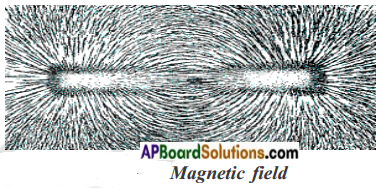Take a bar magnet and place it on a table. Place a thick white paper over it.
On the paper, sprinkle fine powder of iron as shown in the figure. Tab the table or the paper gently with pen/pencil.
a) Do you find the pattern of iron filings there?
Yes.

b) Rotate the magnet in different directions and do the same. How has the pattern changed?
In different directions that we will observe different patterns.

c) Why the iron filings set pattern around magnet?
Iron filings set themselves in a pattern because of magnetic field created by the magnet.

d) What is the space around the magnet called?
The space around magnet where its influence can be detected is called magnetic field.Activity – 5

Question 5.
Preparing a list of examples for muscular force:
List at least ten activities where we apply muscular force to perform various tasks in table.

 List of activities where we exert force Lowering a basket Lifting a bucket from a well Pushing a car Pulling a cow Opening a drawer Opening or shutting a door Hitting a cricket ball with a bat Playing badminton Digging a bore well Raising a flag

Activity – 6

Question 6.
Observing the changes in any muscle while working:
Do you feel your muscle get tightened while performing a physical activity? What could be the reason for it?
Take a dumbbell and lift it in different ways and observe while doing this exercise which muscle is going to be shortened.
The term muscles refer to multiple bundles of muscle cells held together. Muscles are normally arranged in such a way that, as one group of muscles contract or shortens, another group relaxes or expands. So we feel the muscles get tightened while performing any physical activity.
Force of friction:
When you roll a ball on a level ground it invariably stops after sometime.
a) Why does the ball stop?
Due to some opposing force.

b) Is there any hidden force which brings it to stop?
Frictional force between floor and ball.

c) If you stop peddling your bicycle on a level road you observe that its speed decreases gradually. Why does the speed of bicycle decrease gradually? Is there any force acting on it which tends to reduce its speed?
The speed of bicycle decreases gradually due to some opposing force. The force is frictional force between road and tyre.Activity – 7

Question 7.
Observing the motion of a ball on different surfaces.
Try to roll a ball on different surfaces like carpet, rough roads, smooth floor, etc. On which surface does the ball roll farther ?
On the smooth floor it roll farther the reason is the force of resistance to the motion seems to be more on the rough surfaces than on the smooth surfaces.

Activity – 8

Question 8.
Observing the motion of objects on an inclined plane:
Take a tray. Place a small ice cube, eraser and a rupee coin to stay on a line at one end of the tray. Now slowly lift this end of the tray as shown below.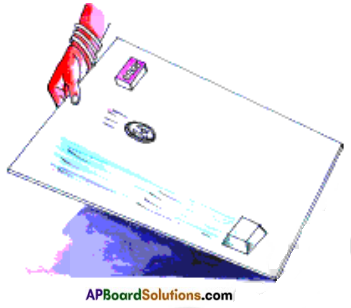a) What do you observe?
The three objects tends to slide downwards.

b) Which of these three objects slides down first? Why?
Ice cube rolls first because the melting of ice takes place
and water acts as lubricant and decrease the frictional force.

c) Do all objects experience the same resistance to motion? If not why?
No, all of them does not experience same resistance in motion because the irregularities present in the surfaces of the objects are different.

d) Which of the objects experiences more resistance to motion? Why?
The eraser experiences more frictional force due to more normal force exerted on the surface of eraser.e) Why the change in resistance experienced by the objects though they all slide down on the same plane?
The frictional force depends on nature of surface. So different surfaces exerts differed frictional forces, therefore there is a change in resistance.

f) Did you ever experience slipping on a floor?
Yes.

g) What conditions caused you to slip?
When we walk on a smooth floor.

h) Did you experience slipping while you are walking on wet mud?
Yes.

i) Why do most road accidents happen during rainy days?
Rain water acts as lubricant which decrease the frictional force between tyre and road thereby vehicles slip more due to that accidents occur more on rainy days.

j) Would it be possible to drive a car if there were no friction between the tyres of car and the road?
No, it is not possible.
Normal force:

k) Place your science book on the table. Is it in a state of rest?
Yes.

l) Is there any force acting on that book?
Yes.

m) Imagine that the table has disappeared suddenly by magic. What will happen then?
The book will fall down due to gravitational pull of the earth.

n) When a book is lying on the table, the gravity pulls the book down all the time but it does not fall down. Why?
It is supported by the table.

o) What is the force which supports against gravity by pushing it upward called?
The force that a solid force exerts on any object in the normal direction is called the normal force.
Tension:
As shown in the figure a stone is suspended with the help of a thread and its free end is tied to the ceiling.

p) What is the state of the stone?
The stone is at the state of rest.

q) What are the forces acting on it?
Gravitational force acts downwards and the force exerted by string acts upwards.r) What will happen if the thread is broken?
The stone will fall downwards due to gravitational pull.

s) For a stone tied to the thread there exists a force which supports the stone against gavity by pulling upwards. What do we call this force?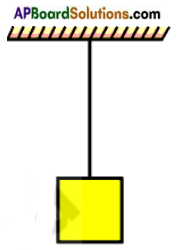It is called tension.

t) What is tension?
When we stretch a rope or a thread the tightness of rope or thread is called tension. Tension is a contact force.

Lab Activity

Write an activity to measure limiting force beared by a thread:
(OR)
Conduct an experiment to find the limiting force that can be borne by different threads.
Aim: To find the limiting force that can be borne by a thread.
Material used: Spring balance, weights, light threads, weight hanger.
Procedure:
i) Arrange the system as shown in figure. Put some small weights like 50 gm on the weight hanger and note the readings of the spring balance. Now, add some more weights to the hanger and note the readings of spring balance. Do the same till the thread is broken.ii) Separate the whole system from the ceiling, and tie the thread to weight hanger and now slowly pull up the whole system with your hand when there is a small weight on the hanger.
Note down the readings of the balance when the thread is broken that give limiting force beared by a thread.

a) What do you observe from the readings when it pulled up and released to move down?
We will observe from the readings that another force is worked on this system when it is pulled up and released move down.

b) Is the thread broken when the whole system is pulled quickly up?
No, the thread is not broken when the whole system is pulled quickly up.Net force:
a) What are the forces acting on an object placed on a floor?
Gravitational force (vertically down) and normal force (vertically up).

b) Do you observe any change in the state of rest of that object, because of these forces and why?
No, because these forces acting on the object are equal and opposite in direction. Hence there is no change in its state.

c) What is the net force acting on object in this case?
Zero.
Imagine that the same object is kept in a lift which is accelerating.

d) How many forces are acting on this object?
Weight of the object (vertically down) and accelerating force (vertically up).

e) Is the net force acting on the object zero? Why?
No, it is not equal to zero because the object is moving upward with non-uniform motion.

Activity – 9

Question 9.
Effects of net force acting on a table :
a) Try to push a heavy wooden table. Is it hard to push ?
Yes.

b) Ask your Mend to help in pushing the table in the same direction, as shown in the figure. Do you find it easier to move the table now? Why?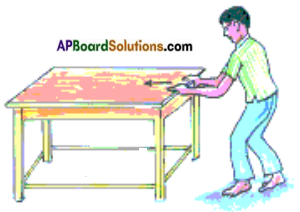Yes, because both of us applying force in the same direction on the table. So resultant applied force increases so it is easier to move the table.

c) Now ask your Mend to push the table from the opposite side as shown in the figure.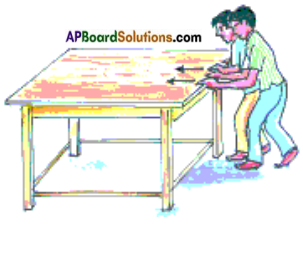Does it move ? If it moves, then in which direction does it move?
If both of us apply same force with equal magnitude the table does not move.
If it moves, it moves in the direction where there is larger force is applied.d) If we apply two unequal forces F1, F2 applied in the same direction and opposite direction (F1 > F2) then what is the net force ?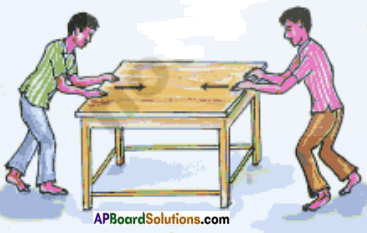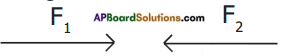If F1, F2 act in same direction, net force = F1 + F2
If F1, F2 act in opposite direction, net force = F1 + (-F2) = F1 – F2

Activity – 10

Question 10.
Effects of stretched rubber bands on fingers:
Take a rubber band, stretch it using your fingers. When you stretch the rubber band it exerts force on your fingers and you feel the force of pull on your fingers.
a) What happens if you add one more similar rubber band around your fingers and stretch both together to the same length ? Do you feel the combination of two bands exerts a larger force than that of one?
Yes, the net force is the combination of two forces. So they exerts larger force.

b) Let us say the force exerted by one rubber band is F units and the force exerted by the second rubber band is also F units. Then what will be the net force of two rubber bands?
Net force (Fnet) = F + F
= 2F units

c) What is the net force acting on your finger when three, four, etc. rubber bands are used?
If three rubber bands are used the net force = F + F + F = 3F
If four rubber bands are used the net force = F + F + F + F = 4F

How to calculate net force from free body diagrams:
Let a car moving with non-uniform speed along a road.
What are the forces acting on the car? What is the net force acting along the vertical direction? What is the net force acting along horizontal direction? Draw all the relevant forces acting on the body by using free body diagram.
Choose a coordinate system with X and Y axis in figure. Sign convention is to be taken along X and Y directions. Add forces algebraically with sign conventions along X and Y axis respectively.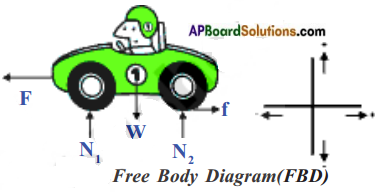The forces acting on the car are, shown in FBD (Free body diagram).
They are Force applied by the engine = F
Friction applied by road = f
Normal forces are N1 and N2.
Gravitational force (Fg) = W
∴ Net force along X – direction,
Fnet, x = f – F
(∵ They act in opposite direction)
Net force along Y – direction,
Fnet, y = (N1 + N2) – W
(∵ They act in opposite direction)Activity – 11

Question 11.
Effect of force on the direction of motion and state of the body:
Place a football on the ground. The ball will remain in the state of rest unless someone kicks the ball.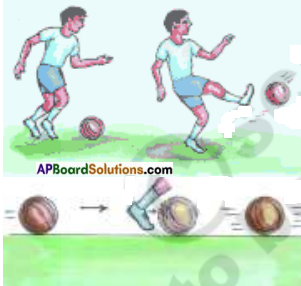a) Kick the ball what happens? Does the ball start moving?
Yes, the ball start moving

b) Kick the moving ball again in the same direction.
What will be the result?
The speed of the ball increases.

c) Place your hand or leg against the ball. Does the ball stop ?
Yes. You might have seen children playing with a rubber tyre by pushing it with a stick. They push the tyre again and again with the stick to increase its speed.

d) Do you understand why the speed of the tyre increases whenever it is pushed by the stick?
With every push they are applying a little more force on the moving tyre in the direction of motion. Hence speed of the tyre increases continuously.

e) What happens if net force acts opposite to the motion of an object ?
The net force acts opposite to the direction of motion of an object then it either slows down the object or brings it to a stop.

f) Give some more examples where the object speeds up or slows down or a change may occur in its direction of motion, when we exert a force on it?
If wind blows in the direction of motion of bicycle, the speed of bicycle increases. If wind blows in the opposite direction of motion of bicycle, the speed of bicycle decreases.

Activity – 12

Question 12.
Effects of net force on direction of moving object: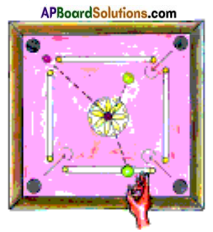a) Hit a carrom coin with the striker. Ask your friends to do the same. Does the coin move in the same direction in each case ?
If not why?
No, you can observe that when you hit the coin with the striker not only does the coin changes its direction, but the striker changes its direction too.

b) What might be the cause of that?
The net force makes a stationary object move and also changes the speed and direction of moving object.

c) Does the force change only the state of motion ? Are there any other effects of force?
No, the force changes the direction.Activity – 13

Question 13.
Effects of force on the shape of an object:
In the given table some situations are given in the first column showing how the force is applied on an object. Observe the shape of the objects carefully before and after applying the force. Mark T for temporary change and ‘P’ for permanent change.

 Action of force Change in shape (temporary / permanent) Stretching rubber band Temporary Squeezing sponge Temporary Tearing paper Permanent Crushing plastic bottle Permanent Making chapathi Permanent Breaking glass Permanent

Activity – 14

Question 14.
Change in effect of force with area of contact:
a) Take a pencil. Just push its rounded end on your palm. Now push from the other side of the pencil gently so that the sharp end is on your palm. What difference did you experience? Why?
It applies more force when sharp end touches the palm.

b) Why do the porters place turbans (talapaga) on their head when they have to carry heavy loads?
The reason is, they are increasing surface area there by they are decreasing the force applied by object.

c) Why do school bags and shopping bags have broad straps as handles?
As the surface area increases the force applied by object decreases.

d) Have you ever wondered why the lorries carrying loads have a large number of broader tyres?
The effect of force depends on the area of contact on which the force is acting. When the area of contact is more then the force acting is less. So lorries have a large number of broader tyres to carry heavy loads.

Activity – 15Question 15.
Identifying effects of force:
Take two trays. Fill both the trays with lime powder or fine sand. Now take two rectangular bricks of equal mass and similar shape. Keep one brick vertically in one tray and the other brick horizontally in the second tray.
a) Do both bricks sink to the same depth in lime powder? If not why?